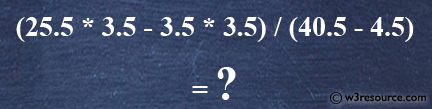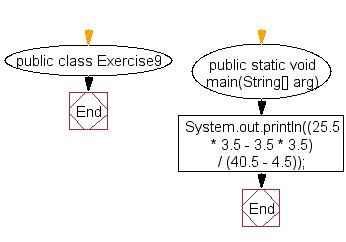﻿ Java - Display and compute specified expressions

# Java: Display and compute specified expressions

## Java Basic: Exercise-9 with Solution

Write a Java program to compute the specified expressions and print the output.

Specified Expression :
(25.5 * 3.5 - 3.5 * 3.5) / (40.5 - 4.5)

Pictorial Presentation:Sample Solution:

Java Code:

``````public class Exercise9 {

public static void main(String[] arg) {
// Calculate and print the result of a mathematical expression
System.out.println((25.5 * 3.5 - 3.5 * 3.5) / (40.5 - 4.5));
}
}
```
```

Explanation:

The above Java code calculates and prints the result of the following mathematical expression:

(25.5 * 3.5 - 3.5 * 3.5) / (40.5 - 4.5)

The code performs arithmetic operations, including multiplication, subtraction, and division, and displays the final result. In this case, the result will be printed to the console.

Sample Output:

```2.138888888888889
```

Flowchart:Java Code Editor:

What is the difficulty level of this exercise?

Test your Programming skills with w3resource's quiz.

﻿